##### The Japanese Bonsai specialist
Direct order Contact Help / Services Newsletter# Shelf to display

Himeshara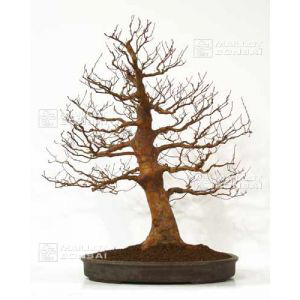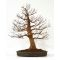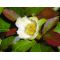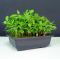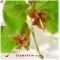ref. : 5294

6,00

This item is temporarily unavailableNotify availability

###### Description

Sachet of + - 60 very cool seeds of Japan of stewartia monadelpha. Family of the camellia requiring a soil humifère and an exhibition mid-shades. Deciduous. Remarkable flowering cream-colored white occurring again all the summer. Beautiful colors autumnal orange rose. Bush to trained port. Red peel exfoliating. Leaves oval, green clear, finely toothed. Flowers in the shape of cut, pure white, to orange stamens yellow. Instructions for use include in the sachet. The seedling takes place in terrine after having placed the seeds in a plastic sachet with the cool sand in the ferry to vegetables of the refrigerator and this during minimum 2 months. Then then to make the seedling in one soil draining to ambient temperature. Raised in 60/80 days. Recording in individual pot at the end of 1ere year. To avoid the excesses of water hot Stratification to 20°C as possible during 3 months. Rusticity: Very rustic in full earth until -15°C and more asks a protection at the time of his/her/its culture in bonsaï.

#seeds 6.5 #monadelpha 4 #stewartia 3.9 #sachet 3.6 #more 3.4 #seedling 2.8 #orange 2.6 #during 2.6 #months 2.6 #white 2.5

Formule
(( ROUND((CHAR_LENGTH(b.article_nom)-CHAR_LENGTH(REPLACE(b.article_nom, 'seeds', '')))/LENGTH('seeds')) + ROUND((CHAR_LENGTH(b.article_description)-CHAR_LENGTH(REPLACE(b.article_description, 'seeds', '')))/LENGTH('seeds')) ) * 4.5) + (( ROUND((CHAR_LENGTH(b.article_nom)-CHAR_LENGTH(REPLACE(b.article_nom, 'monadelpha', '')))/LENGTH('monadelpha')) + ROUND((CHAR_LENGTH(b.article_description)-CHAR_LENGTH(REPLACE(b.article_description, 'monadelpha', '')))/LENGTH('monadelpha')) ) * 4) + (( ROUND((CHAR_LENGTH(b.article_nom)-CHAR_LENGTH(REPLACE(b.article_nom, 'stewartia', '')))/LENGTH('stewartia')) + ROUND((CHAR_LENGTH(b.article_description)-CHAR_LENGTH(REPLACE(b.article_description, 'stewartia', '')))/LENGTH('stewartia')) ) * 3.9) + (( ROUND((CHAR_LENGTH(b.article_nom)-CHAR_LENGTH(REPLACE(b.article_nom, 'sachet', '')))/LENGTH('sachet')) + ROUND((CHAR_LENGTH(b.article_description)-CHAR_LENGTH(REPLACE(b.article_description, 'sachet', '')))/LENGTH('sachet')) ) * 3.6) + (( ROUND((CHAR_LENGTH(b.article_nom)-CHAR_LENGTH(REPLACE(b.article_nom, 'seedling', '')))/LENGTH('seedling')) + ROUND((CHAR_LENGTH(b.article_description)-CHAR_LENGTH(REPLACE(b.article_description, 'seedling', '')))/LENGTH('seedling')) ) * 2.8) + (( ROUND((CHAR_LENGTH(b.article_nom)-CHAR_LENGTH(REPLACE(b.article_nom, 'during', '')))/LENGTH('during')) + ROUND((CHAR_LENGTH(b.article_description)-CHAR_LENGTH(REPLACE(b.article_description, 'during', '')))/LENGTH('during')) ) * 2.6) + (( ROUND((CHAR_LENGTH(b.article_nom)-CHAR_LENGTH(REPLACE(b.article_nom, 'months', '')))/LENGTH('months')) + ROUND((CHAR_LENGTH(b.article_description)-CHAR_LENGTH(REPLACE(b.article_description, 'months', '')))/LENGTH('months')) ) * 2.6) + (( ROUND((CHAR_LENGTH(b.article_nom)-CHAR_LENGTH(REPLACE(b.article_nom, 'orange', '')))/LENGTH('orange')) + ROUND((CHAR_LENGTH(b.article_description)-CHAR_LENGTH(REPLACE(b.article_description, 'orange', '')))/LENGTH('orange')) ) * 2.6) + (( ROUND((CHAR_LENGTH(b.article_nom)-CHAR_LENGTH(REPLACE(b.article_nom, 'white', '')))/LENGTH('white')) + ROUND((CHAR_LENGTH(b.article_description)-CHAR_LENGTH(REPLACE(b.article_description, 'white', '')))/LENGTH('white')) ) * 2.5) + (( ROUND((CHAR_LENGTH(b.article_nom)-CHAR_LENGTH(REPLACE(b.article_nom, 'soil', '')))/LENGTH('soil')) + ROUND((CHAR_LENGTH(b.article_description)-CHAR_LENGTH(REPLACE(b.article_description, 'soil', '')))/LENGTH('soil')) ) * 2.4)

## Secure payment## Delivery

Our logistic partners :